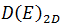# Density of states in 1D, 2D, and 3D - Engineering physics

Density of states in 1D, 2D, and 3D

In 1-dimension

The density of state for 1-D is defined as the number of electronic or quantum states per unit energy range per unit length and is usually denoted byWhere dN is the number of quantum states present in the energy range between E and E+dE

Here factor 2 comes because each quantum state contains two electronic states, one for spin up and other for spin down.

Equation (1) can be written as

From eq. (2), we have

As we know

So

By using Eqs. (4)and (5), eq. (3) becomes

This result is shown plotted in the figure.

It shows that all the states up to Fermi-level  are filled at 0K.

In 2-Dimensional

The density of state for 2D is defined as the number of electronic or quantum states per unit energy range per unit area and is usually defined asArea (A)Here factor 2 comes because each quantum state contains two electronic states, one for spin up and other for spin down.

Eq. (7) can be written as

1.1.